# How to☝️ Use the FIND Function in Google Sheets

To use the FIND function in Google Sheets, select the blank cell where you need your result, navigate to the Formula bar, type the formula =FIND(“a”, A2, 1), and press Enter

Read the article below so you don’t miss any important information on this topic—and learn how to make the best use of this function!

## How to Quickly Apply the FIND Function in Google Sheets

Learn out how to use this function in no time at all!

Difficulty: Beginner

Time Estimate: 5 seconds

1. Click on a blank cell.
2.  Go to the Formula bar and enter the formula =FIND(“a”, A2, 1). In our case, a is the element we are looking for, A2 is the cell reference, and 1 indicates where to begin the search.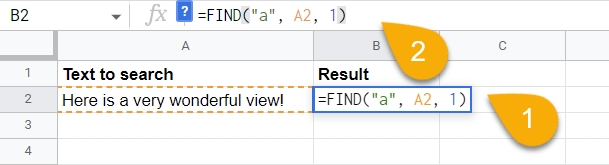3. Hit the Enter key on your keyboard.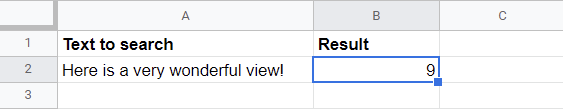Congratulations! Here is the result.

## Drilling Down into the FIND Function

### Description

The FIND function is a built-in function in Google Sheets that allows you to search for data in your spreadsheet. It can be used to find data in a single column or across multiple columns. The FIND function is case-sensitive.

Suppose you have data where you need to find a specific value. The FIND function will help you do that! Simply apply the formula =FIND(“a”, A2, 1).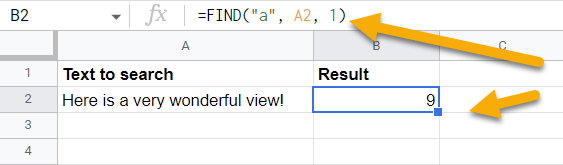### Purpose

The FIND function in Google Sheets is a quick way to locate specific data within your spreadsheet. This function can be used to search for text, numbers, or even cell references.

### Syntax

=FIND(search_for, text_to_search, [starting_at])

### Arguments

• search_for is the text you need to find.
• text_to_search is a cell reference, or cell range, where you want to find the text value.
• starting_at is a value that shows which character to start the search from. As a rule, this is not a required argument and can be avoided, at which point your search will automatically start from the first character.

### Return Value

If you use the FIND function, you will be able to find the position of the element you are searching for.

### Usage Notes

You may encounter the #VALUE! or #ERROR! errors while using the FIND function. There are several reasons for this:

1. You entered an incorrect value in the formula.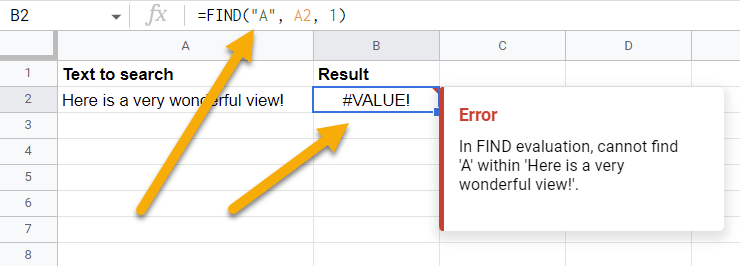This shows that the FIND function is case-sensitive when it comes to searching. In order to make the formula work, you should only use values that are exact.

1. The arguments you used were not presented in the correct order.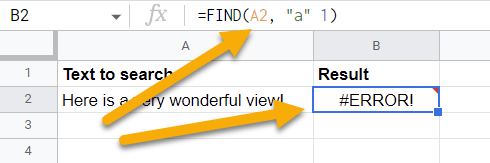Arguments should be arranged as follows: =FIND(search_for, text_to_search, [starting_at]).

Besides those, there are a few other things to note:

1. It is also possible to omit the starting_at argument. If you skip it, you will start the search from the very beginning.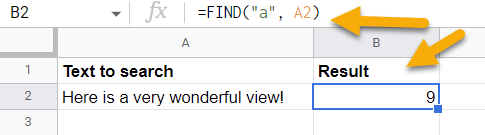1. You can also choose a reference with the value you need to find instead of specifying a text value separately in the formula.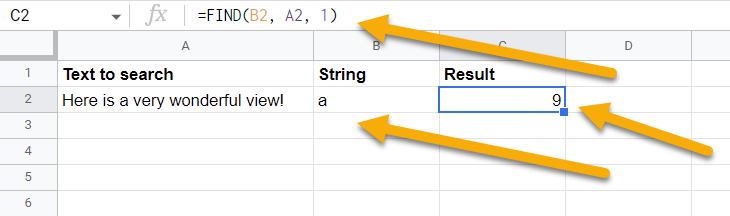### Examples

1. Using the FIND function, enter any text value you need to find.

=FIND(“a”, A2, 1) // Output: 91. Alternatively, you can use the cell reference of the cell containing the text value that you are looking for.

=FIND(B2, A2, 1) // Output: 9## What Is the Practical Use of the FIND Function?

There is no doubt that navigating a large spreadsheet without the use of the FIND function can be a difficult task.

The FIND function is particularly useful for finding a specific value in a large dataset. The FIND function can also be used to locate a specific piece of data in a range of cells. So, this can be extremely helpful when you are trying to analyze data or find trends.